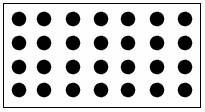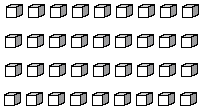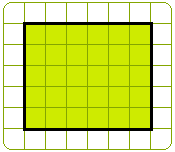Definition Of Array

An Array is an arrangement of a set of numbers or objects in rows and columns.

An array is normally used to write multiplication and division number sentences.
A multiplication array can easily be expressed as a repeated addition.
A division array can easily be expressed as a repeated subtraction.

Examples of ArrayThe dots in the above figure are arranged in 4 rows and 7 columns. It can also be written as 7 × 4 = 7 + 7 + 7 + 7 = 28The cubes in the above figure are arranged in 4 rows and 9 columns. It can also be written as 9 × 4 = 9 + 9 + 9 + 9 = 36.

Solved Example on Array

Ques: Greg shaded the cells on a grid to make a rectangle, as shown. Which number sentence could be used to determine the total number of cells shaded to make the rectangle?A. 4 × 6 = 24
B. 1 × 25 = 25
C. 5 × 6 = 30
D. 30 × 3 = 90